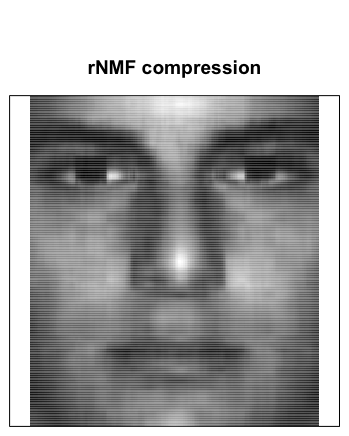This page provides installation instruction and usage examples for the R package rnmf.

## Robust nonnegative matrix factorization (rNMF)

rNMF decomposes a p by n nonnegative matrix $$X$$ into $$X\approx WH$$ by minimizing a penalized objective function

$||X-WH||_{\gamma}^2 + \alpha||W||_F + \beta\sum||H_{.j}||_1^2$

Here rows of $$X$$ are variables and columns are observations. Both $$W$$ and $$H$$ are nonnegative. $$W$$ is p by k, $$H$$ is k by n with $$k<\min\{p,n\}$$. $$\alpha$$ and $$\beta$$ control the magnitude of $$W$$ and the sparsity of $$H$$.

As a major advantage over the regular NMF, rNMF detects outliers in $$X$$ and remove them from the fitting objective function. See [J. Sun, Y. Xu, K. Lopiano and S. Young 2013] for more details.

The procedure can be used to extract clean low dimensional structure from corrupted high dimensional data, such as images, DNA/RNA sequencing data and documents. It can also be used to compress a single corrupted image.

## Install and load the package

After downloading the package file “rnmf_0.5.tar.gz” from [GitHub repo address here], put it in your preferred working directory and run both of the following lines (remove the ‘##’ from the first line):

## install.packages("rnmf_0.5.tar.gz", repos = NULL, type = "source")
library(rNMF)

## Examples

rNMF can be used on any nonnegative data matrix. For illustration purposes, we give two examples with image data.

The first example demonstrates the decomposition and outlier extraction of multiple corrupted images by rNMF. The second example demonstrates the compression of a single corrupted image by rNMF.

### Example 1

First load the build-in data set ‘Symbols_c’, a 5625 by 30 matrix where each column contains a vectorized 75 by 75 image.

data(Symbols_c)

The function ‘see()’ shows the corrupted images:

see(Symbols_c, title = "Corrupted data set")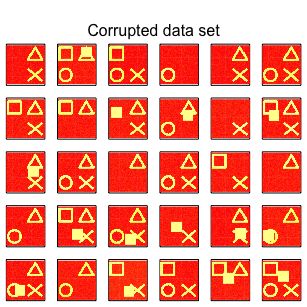Solid boxes in the images are corruptions. In the following we compare the decomposition results by regular NMF and rNMF.

Regular NMF decomposition (gamma = FALSE, k = 4) gives the following result.

res <- rnmf(Symbols_c, k = 4, showprogress = FALSE, my.seed = 100)
## Done. Time used:
##    user  system elapsed
##   4.545   0.084   4.739
## No trimming.
## Input matrix dimension: 5625 by 30
## Left matrix: 5625 by 4. Right matrix: 4 by 30
## alpha = 0. beta = 0
## Number of max iterations = 20
## Number of iterations = 16

The class of res is “rnmf”(see ?rnmf for details). res$fit gives the reconstruction: see(res$fit, title = "Regular NMF reconstruction with k = 4")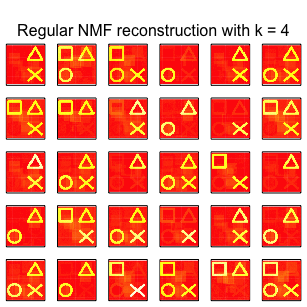Basis vectors are given by res$W see(res$W, title = "Regular NMF basis", layout = c(1,4))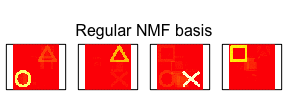Shadows in above images indicate that corruptions were not removed and contaminated the decomposition.

Now the same data is decomposed with rNMF with trimming (gamma = 0.03, k = 4)

res2 <- rnmf(Symbols_c, k = 4, gamma = 0.03, showprogress = FALSE,
my.seed = 100, tol = 0.0001, maxit = 50)
## Done. Time used:
##    user  system elapsed
##  23.191   0.555  24.411
##
##  Trimming mode = "cell". Proportion trimmed: 0.03
## Input matrix dimension: 5625 by 30
## Left matrix: 5625 by 4. Right matrix: 4 by 30
## alpha = 0. beta = 0
## Number of max iterations = 50
## Number of iterations = 23

rnmf reconstruction:

see(res2$fit, title = "rNMF reconstruction with k = 4")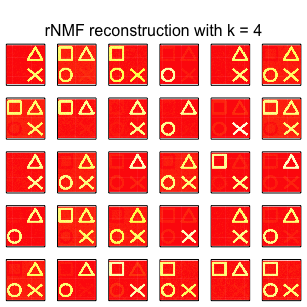see(res2$W, title = "rNMF basis vectors", layout = c(1,4))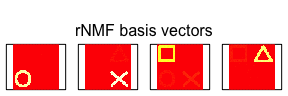The results are more clear and the outliers are also extracted:

outliers <- matrix(0, nrow = nrow(Symbols_c), ncol = ncol(Symbols_c))
outliers[res2$trimmed[[res2$niter]]] <- 1
see(outliers, title = "Outliers extracted by rNMF")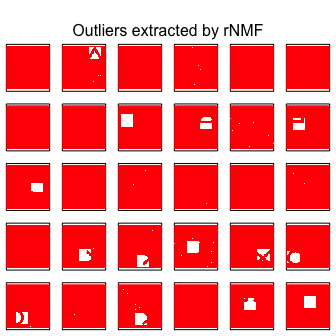### Example 2

In this example we compare the compression of a single corrupted image by both regular NMF and rNMF. First we load a build-in corrupted face image.

data(face)

First let’s look at the corrupted face image:

see(face, title = "Corrupted face image", col = "grey", input = "single")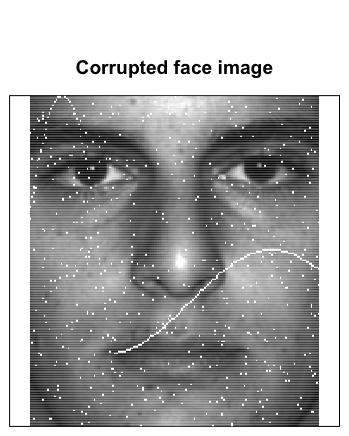#### Regular NMF compression (trim = FALSE)

res <- rnmf(face, k = 10, showprogress = FALSE, my.seed = 100)
## Done. Time used:
##    user  system elapsed
##   0.285   0.006   0.296
## No trimming.
## Input matrix dimension: 192 by 168
## Left matrix: 192 by 10. Right matrix: 10 by 168
## alpha = 0. beta = 0
## Number of max iterations = 20
## Number of iterations = 8
see(res$fit, title = "NMF compression", col = "grey", input = "single")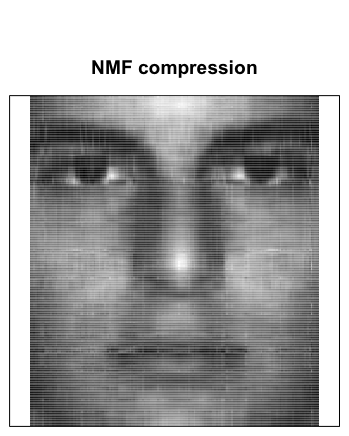The compression is heavily contaminated. #### rNMF compression with trimming res2 <- rnmf(face, k = 10, gamma = 0.025, showprogress = FALSE, my.seed = 100) ## Done. Time used: ## user system elapsed ## 0.927 0.014 0.974 ## ## Trimming mode = "cell". Proportion trimmed: 0.025 ## Input matrix dimension: 192 by 168 ## Left matrix: 192 by 10. Right matrix: 10 by 168 ## alpha = 0. beta = 0 ## Number of max iterations = 20 ## Number of iterations = 7 see(res2$fit, title = "rNMF compression", col = "grey", input = "single")